Courses

Electrostatic MCQ (With Solution) Level - 1: Test 1

30 Questions MCQ Test | Electrostatic MCQ (With Solution) Level - 1: Test 1

Description
Attempt Electrostatic MCQ (With Solution) Level - 1: Test 1 | 30 questions in 60 minutes | Mock test for Class 12 preparation | Free important questions MCQ to study for Class 12 Exam | Download free PDF with solutions
QUESTION: 1

Solution:
QUESTION: 2

Two identical conducting balls A and B have positive charges q1 and q2 respectively but. The balls are brought together so that they touch each other and then kept in their original positions. The force between them is

Solution:

Original charges on spheres A and B be q1 and q2 respectively.
Distance between the two spheres = r
Since, both the spheres are of same size, they will possess equal charges on being brought in contact.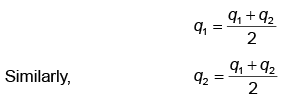Therefore, new force of repulsion between spheres A and B is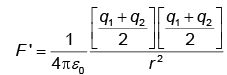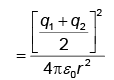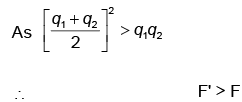QUESTION: 3

Under the action of a given coulom bic forc e the acceleration of an electron is 2.5×1022 m/s2. Then the magnitude of the acceleration of a proton under the action of same force is nearly

Solution:

Coulombic force on electron, Fe = aeme
Similarly, for proton Fp = apmp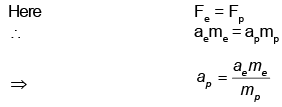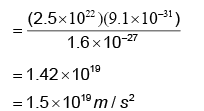QUESTION: 4

A conductor has been given a charge -3×10-7C by transferring electrons. Mass increase (in kg) of the conductor and the number of electrons added to the conductor are respectively.

Solution:

Number of electrons added to the conductor
n = q/e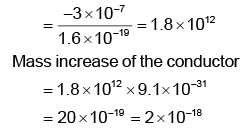QUESTION: 5

What is the total charge in coulomb of 75.0 kg of electrons?

Solution:

We know that m = 9.11×10-31 kg and M = 75.0 kg
So,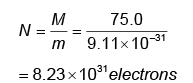Hence, total charge on the collections is q = -Ne
= -(8.23 × 1031 )(1.60 × 10-19 )
= 1.32 × 1013 C

QUESTION: 6

Under the influence of the coulomb field of charge +Q, a charge - q is moving around it in an elliptical orbit.Find out the correct statements(s).

Solution:

The force always acts along the r, So torque will always be zero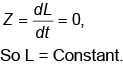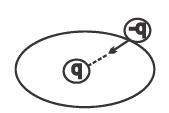QUESTION: 7

A charge Q is placed at each of the opposite cor n ers of a square. A charge q is placed at each of the other two corners. If the net electrical force on Q is zero, then the Q/q equals.

Solution:

Three forces F41, F42and F43 acting on Q as shown resultant of F41 + F43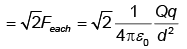Resultant on Q becomes zero only when q charges are of negative nature.
⇒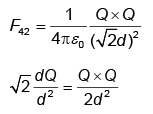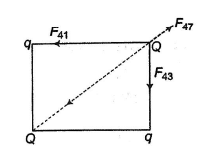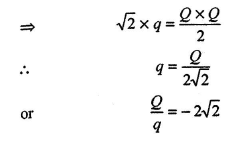QUESTION: 8

An object A has a charge of -2 µC and the object B has a charge of +6µC. Which statement is true?

Solution:

Vector from of Coulomb's law states that FAB are equal and opposite.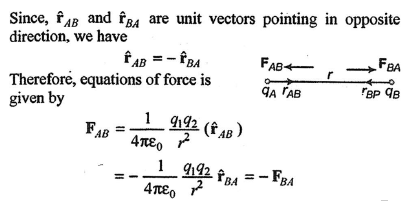QUESTION: 9

A charge of 1 µC is divided into two parts such that their charges are in the ratio of 2 : 3. These two charges are kept at a distance 1 m apart in vacuum. Then, the electric force between them (in N) is

Solution:

Ratio of charges = 2 : 3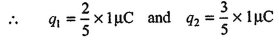Electrostatic force between the two charges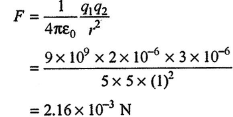QUESTION: 10

Two identical positive charges are fixed on the y-axis at equal distances from the origin O. A negatively charged particle starts on the x-axis, at a large distance from O, moves along the x-axis, passes through O and moves far away from O. Its acceleration A is taken as positive along its direction of motion. The best graph between the particle's acceleration and its x-coordinate is represented by

Solution:

Let two identical positive charges (+q) are placed on both sides at a distance a from the origin O and a negative charge (-q') is placed at a large distance x from O

QUESTION: 11

Two identical metal spheres charged with +12µF and - 8µF are kept at certain distance in air. They are brought into contact and then kept at the same distance. The ratio of the magnitudes of electrostatic forces between them before and after contact is :

Solution: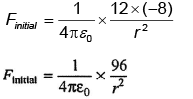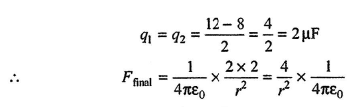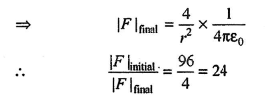QUESTION: 12

The force of interaction between two charges q1 = 6µC and q2 = 2µC is 12 N. If charge q = -2µC is added to each of the charges, then the new force of interaction is

Solution: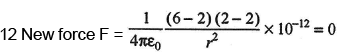QUESTION: 13

In nature, the electric charge of any system is always equal to

Solution:

If the charge of an electron is taken as elementary unit ie, quanta of charge, the charge on any body will be some integral multiple of e ie, q = n e with n = 1, 2, 3...

QUESTION: 14

A comb run through one's dry hair attracts small bits of paper. This is due to

Solution:

When a comb runs through one's dry hair, then comb gets charged and when it comes close to paper, it induces opposite charges in paper. The field due to the charges in comb, polarises the atoms in the paper.
Finally it attracts the paper.

QUESTION: 15

A and B are two identical spherical charged bodies which repel each other with force F, kept at a finite distance. A third uncharged sphere of the same size is brought in contact with sphere B and removed. It is then kept at mid-point of A and B. Find the magnitude of force on C.

Solution:

From Coulomb's law, the force of attraction between two charged particles (q), kept at distance r apart is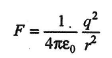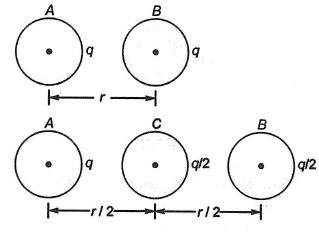When two identical spheres are brought in contact, charge on them is equalised, hence total charge on C is equaly shared when brough in contact with spehere B having a charge q.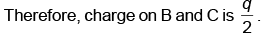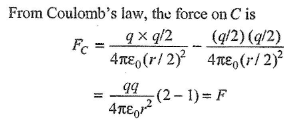QUESTION: 16

Two equal charges as separated by a distnce d. A third charge placed on a perpendicular bisector at x distance from center will experience maximum coulomb force. when.

Solution: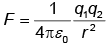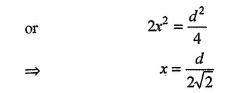Taking the net force, we have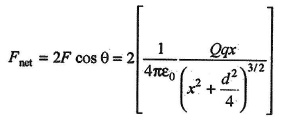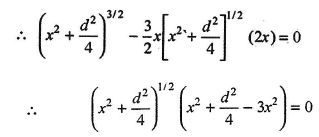QUESTION: 17

Charges are placed at corners of a square of side a as shown in the following figure. The charge A is in equilibrium. The ratio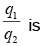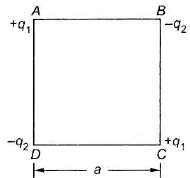Solution:

The charge A experiences three forces F1, F2 and F3 as shown in figure.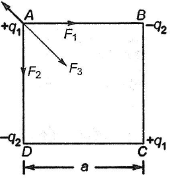Now,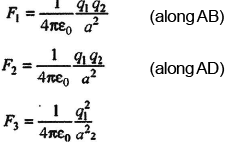The resultant of F1 and F2 should be equal and opposite to F3 to keep the system in equilibrium.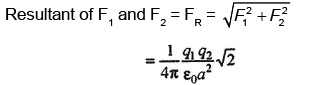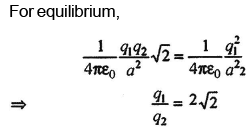QUESTION: 18

Consider a neutral conducting sphere. A positive point charge is placed outside the sphere. The net charge on the sphere is then

Solution:

Charge will be induced in the conducting sphere, but net charge on it will be zero.

QUESTION: 19

A charge Q is divided in two parts q and Q - q. What is value of q for maximum force between them?

Solution:

If r is the distance between q and (Q-  q), the Coulomb force between them is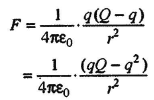For F to be maximum dF/dq = 0 and Q and r are constants.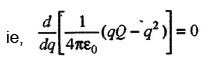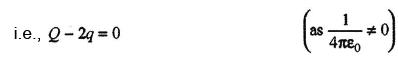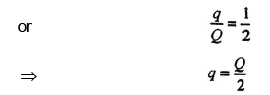QUESTION: 20

Two identical charges repel each other with a force equal to 10 mg wt when they are 0.6 m apart in air (g = 10 ms-2) The value of each charge is

Solution:

Coulomb force is given by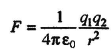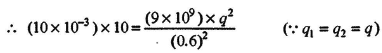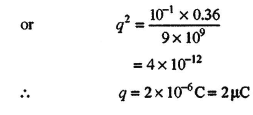QUESTION: 21

Two unit negative charges are placed on a straight line. A positive charge q is placed exactly at the mid-point between these unit charges. If the system of these three charges is in equilibrium, the value of q (in C) is

Solution:

For equilibrium, we have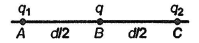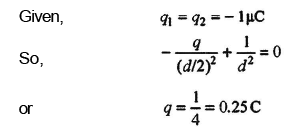QUESTION: 22

Two spherical conductors B and C having equal radii and carrying equal charges in them repel each other with a force F when kept apart at some distance. A third spherical conductor having same radius as that of B but uncharged, is brought in contact with B, then brought in contact with C and finally removed away from both. The new force of repulsion between B and C is

Solution:

Let the spherical conductors B and C have same charge as q.
The electric force between them is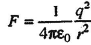r, being the distance between them.
When third uncharged conductor A is brought in contact with B, then charge on each conductor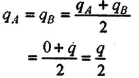When this conductor A is now brough in  contact with C, then charge on each conductor.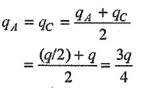Hence, electric force acting between B and C is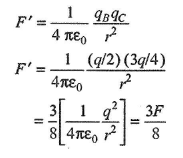QUESTION: 23

Three charges 1µC, 1µC and 2µC are kept at vertices of A, B and C of an equilateral triangle ABC of 10 cm sie respectively, The resultant force of the charge at C is

Solution: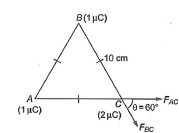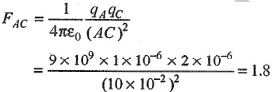Similarly, FBC =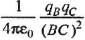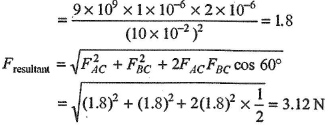QUESTION: 24

Force between two identical charges placed at a distance of r in vacuum is F. Now a slab of dielectric of dielectric constant 4 is inserted between these two charges. If the thickness of the slab is r/2, then the force between the charges will become

Solution: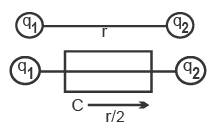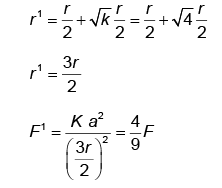QUESTION: 25

Identify the wrong statement in the following. Coulomb's law correctly described the electric force that

Solution:

Coulomb's law is appliable for charged particles, it is not responsible to bind the protons and neutrons in the nucleus of an atom.

QUESTION: 26

If a conducting medium is placed between two charges, then the electric force between them will become

Solution:
QUESTION: 27

A charge q is placed at the centre of the line joining two equal point charges each equal to Q.The system of three charges will be in equilibrium if q is equal to

Solution:

For equilibrium of system of charges, force produced between q and Q + force produced between Q and Q = 0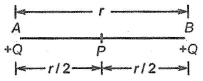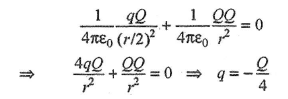QUESTION: 28

An infinite number of charges, each of charge, 1µC, are placed on the x-axis with co-ordinates x = 1, 2, 4, 8, ... ∞. If a charge of 1c is kept at the origin, then what is the net force acting on 1 C charge?

Solution:

From Coulomb's law, the force between two points charges q1,q2 separated by distance r is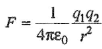The schematic diagram of distribution of charges on xaxis is shown.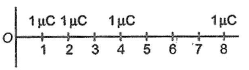The total force acting on 1 C charge is given by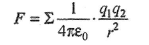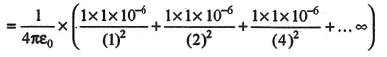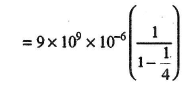Using the fact that sum of GP series is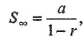where a is first term of series and r thecommon ratio.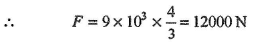QUESTION: 29

Two charges of equal magnitudes and at a distance r exert a force F on each other. If the cahrges are halved and distance between them is doubled, then the new force acting on each charge is

Solution:

From Coulomb's law the force between two points charges q1 and q2, separated by a distance r is given by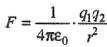Since,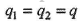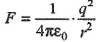In second case when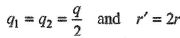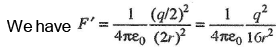Divided Eq. (ii) by Eq. (i), we have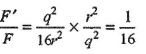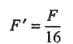QUESTION: 30

When a body is earth connected, electrons from the earth flow into the body. This means the body is

Solution:

Since, the body is connected to earch and the electrons from the earth flow to the body so, it loses its positive charge. Hence, it implies that previously it was positively charged.Use Code STAYHOME200 and get INR 200 additional OFF Use Coupon Code

Track your progress, build streaks, highlight & save important lessons and more!

Similar ContentRelated tests